1-D Kinematics - Lesson 4 - Describing Motion with Velocity vs. Time Graphs

# Determining the Area on a v-t GraphAs learned in an earlier part of this lesson, a plot of velocity-time can be used to determine the acceleration of an object (the slope). In this part of the lesson, we will learn how a plot of velocity versus time can also be used to determine the displacement of an object. For velocity versus time graphs, the area bound by the line and the axes represents the displacement. The diagram below shows three different velocity-time graphs; the shaded regions between the line and the time-axis represent the displacement during the stated time interval.

 The shaded area is representative of the displacement during from 0 seconds to 6 seconds. This area takes on the shape of a rectangle can be calculated using the appropriate equation.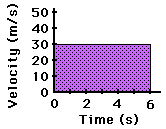The shaded area is representative of the displacement during from 0 seconds to 4 seconds. This area takes on the shape of a triangle can be calculated using the appropriate equation.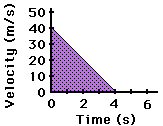The shaded area is representative of the displacement during from 2 seconds to 5 seconds. This area takes on the shape of a trapezoid can be calculated using the appropriate equation.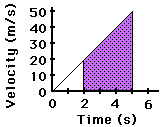The method used to find the area under a line on a velocity-time graph depends upon whether the section bound by the line and the axes is a rectangle, a triangle or a trapezoid. Area formulas for each shape are given below.

 Rectangle Triangle Trapezoid Area = b • h Area = ½ • b • h Area = ½ • b • (h1 + h2)

### Calculating the Area of a Rectangle

Now we will look at a few example computations of the area for each of the above geometric shapes. First consider the calculation of the area for a few rectangles. The solution for finding the area is shown for the first example below. The shaded rectangle on the velocity-time graph has a base of 6 s and a height of 30 m/s. Since the area of a rectangle is found by using the formula A = b x h, the area is 180 m (6 s x 30 m/s). That is, the object was displaced 180 meters during the first 6 seconds of motion.Area = b * h Area = (6 s) * (30 m/s) Area = 180 mNow try the following two practice problems as a check of your understanding. Determine the displacement (i.e., the area) of the object during the first 4 seconds (Practice A) and from 3 to 6 seconds (Practice B).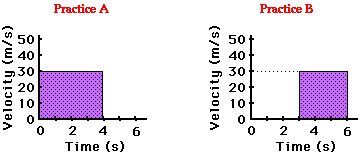### Calculating the Area of a Triangle

Now we will look at a few example computations of the area for a few triangles. The solution for finding the area is shown for the first example below. The shaded triangle on the velocity-time graph has a base of 4 seconds and a height of 40 m/s. Since the area of triangle is found by using the formula A = ½ * b * h, the area is ½ * (4 s) * (40 m/s) = 80 m. That is, the object was displaced 80 meters during the four seconds of motion.Area = ½ * b * h Area = ½ * (4 s) * (40 m/s) Area = 80 mNow try the following two practice problems as a check of your understanding. Determine the displacement of the object during the first second (Practice A) and during the first 3 seconds (Practice B).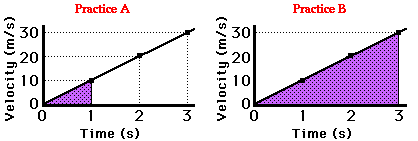### Calculating the Area of a Trapezoid

Finally we will look at a few example computations of the area for a few trapezoids. The solution for finding the area is shown for the first example below. The shaded trapezoid on the velocity-time graph has a base of 2 seconds and heights of 10 m/s (on the left side) and 30 m/s (on the right side). Since the area of trapezoid is found by using the formula A = ½ * (b) * (h1 + h2), the area is 40 m [½ * (2 s) * (10 m/s + 30 m/s)]. That is, the object was displaced 40 meters during the time interval from 1 second to 3 seconds.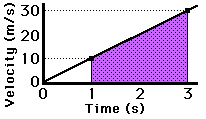Area = ½ * b * (h1 + h2) Area = ½ * (2 s) * (10 m/s + 30 m/s) Area = 40 mNow try the following two practice problems as a check of your understanding. Determine the displacement of the object during the time interval from 2 to 3 seconds (Practice A) and during the first 2 seconds (Practice B).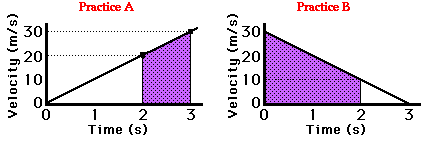### Alternative Method for Trapezoids

An alternative means of determining the area of a trapezoid involves breaking the trapezoid into a triangle and a rectangle. The areas of the triangle and rectangle can be computed individually; the area of the trapezoid is then the sum of the areas of the triangle and the rectangle. This method is illustrated in the graphic below.Triangle: Area = ½ * (2 s) * (20 m/s) = 20 m

Rectangle: Area = (2 s) * (10 m/s) = 20 m

Total Area = 20 m + 20 m = 40 m

It has been learned in this lesson that the area bounded by the line and the axes of a velocity-time graph is equal to the displacement of an object during that particular time period. The area can be identified as a rectangle, triangle, or trapezoid. The area can be subsequently determined using the appropriate formula. Once calculated, this area represents the displacement of the object.

### Investigate!

The widget below computes the area between the line on a velocity-time plot and the axes of the plot. This area is the displacement of the object. Use the widget to explore or simply to practice a few self-made problems.

### We Would Like to Suggest ...Sometimes it isn't enough to just read about it. You have to interact with it! And that's exactly what you do when you use one of The Physics Classroom's Interactives. We would like to suggest that you combine the reading of this page with the use of our Two Stage Rocket Interactive. This Interactive is found in the Physics Interactives section of our website and allows a learner to apply the skill of calculating areas and relating them to displacement values for a two-stage rocket.